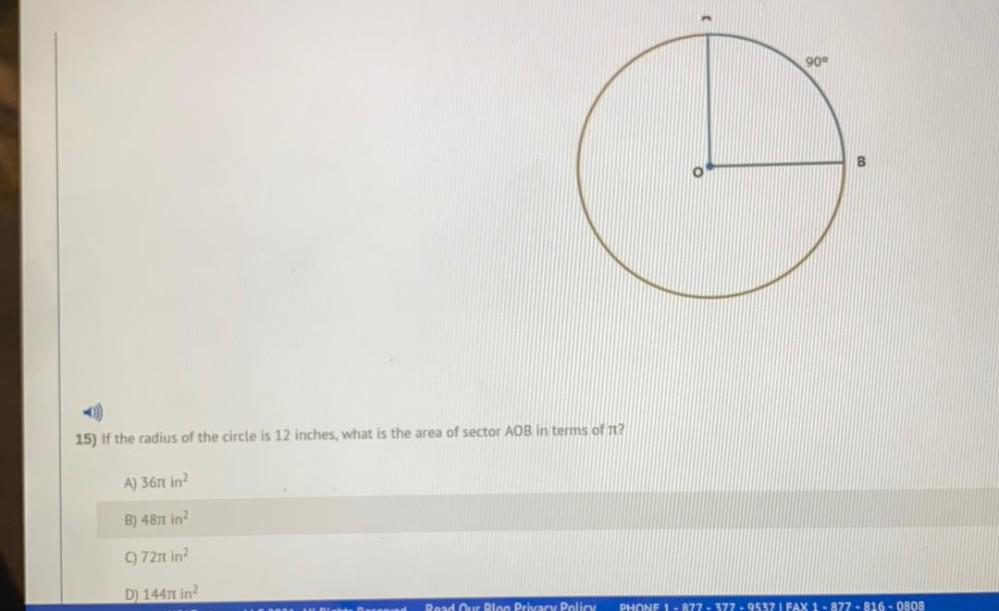Question:

# if the radius of the circle is 12 inches, what is the area of sector AOB in terms of it? given; central angle ,AOB= 90 A) 367 π in^2 B) 487 π in^2 (C)721 π in^2 D) 14471 π in^2if the radius of the circle is 12 inches, what is the area of sector AOB in terms of it? given; central angle ,AOB= 90 A) 367 π in^2 B) 487 π in^2 (C)721 π in^2 D) 14471 π in^2